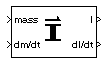# Estimate Inertia Tensor

Calculate inertia tensor

•Libraries:
Aerospace Blockset / Mass Properties

## Description

The Estimate Inertia Tensor block calculates the inertia tensor and the rate of change of the inertia tensor.

Linear interpolation is used to estimate the inertia tensor as a function of mass. The rate of change of the inertia tensor is a linear function of rate of change of mass.

## Ports

### Input

expand all

Mass, specified as a scalar.

Data Types: `double`

Rate of change of mass, specified as a scalar.

Data Types: `double`

### Output

expand all

Inertia tensor, returned as a 3-by-3 matrix.

Data Types: `double`

Rate of change of inertia tensor, returned as a 3-by-3 matrix.

Data Types: `double`

## Parameters

expand all

Gross mass of the vehicle, specified as a double scalar.

#### Programmatic Use

 Block Parameter: `fmass` Type: character vector Values: double scalar Default: `'2'`

Empty mass of the vehicle, specified as a double scalar.

#### Programmatic Use

 Block Parameter: `emass` Type: character vector Values: double scalar Default: `'1'`

Inertia tensor at gross mass of the vehicle, specified as a three-element matrix.

#### Programmatic Use

 Block Parameter: `fI` Type: character vector Values: double scalar Default: `'eye(3)'`

Inertia tensor at empty mass of the vehicle, specified as a scalar.

#### Programmatic Use

 Block Parameter: `eI` Type: character vector Values: scalar Default: `'eye(3)/2'`

## Version History

Introduced before R2006a